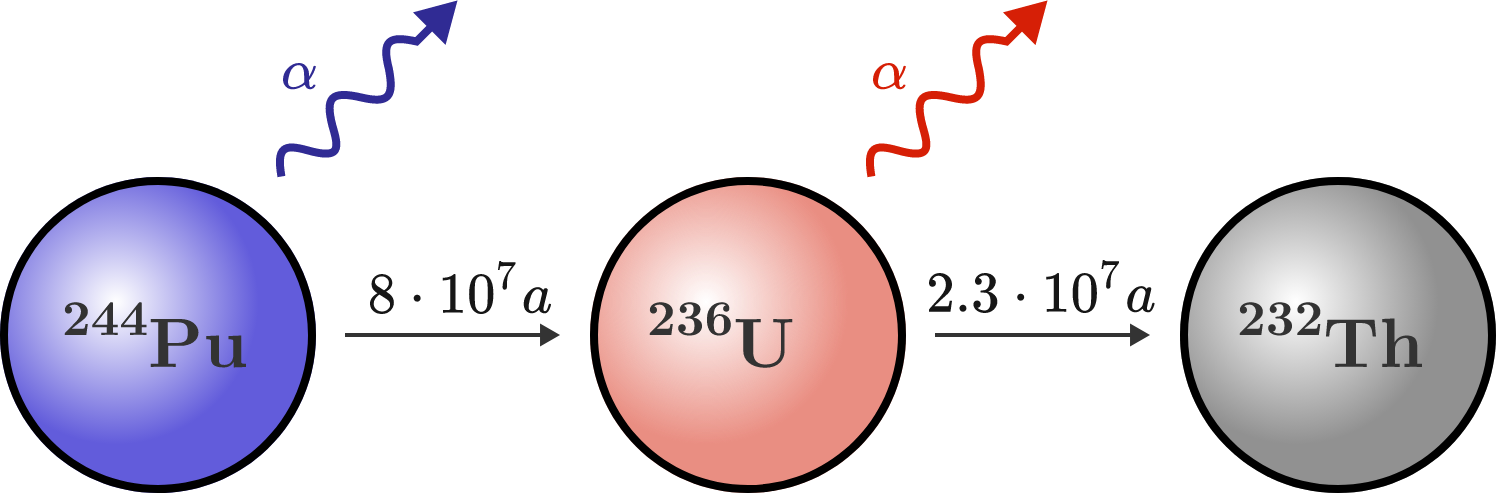# Ancient aliens

NASA has captured an extraterrestrial space probe that has been in space for millions of years. The probe was operated by a kind of radionuclide battery, which on launch consisted of a pure block of plutonium-244. Now, the isotope ratio between thorium-232 and plutonium-244 is 1:1 due to the radioactive decay $(N_\text{Pu} = N_\text{Th}).$

How long was the probe in space? (Specify the time in millions of years and round it to the nearest integer.)Details and Assumptions:

• Plutonium-244 decays in a two-step reaction into thorium-232: $^{244}\text{Pu} \longrightarrow \,^{236}\text{U} \longrightarrow \,^{232}\text{Th}.$
• The half-lives of plutonium-244 and uranium-236 are 80 and 23 million years, respectively.
• Thorium-232 is stable (half-life is longer than 10 billion years).
×

Problem Loading...

Note Loading...

Set Loading...